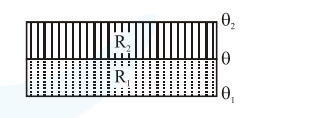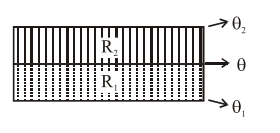# The temperature`
Question:

The temperature $\theta$ at the junction of two insulating sheets, having thermal resistances $\mathrm{R}_{1}$ and $R_{2}$ as well as top and bottom temperatures $\theta_{1}$ and $\theta_{2}$ (as shown in figure) is given by :1. $\frac{\theta_{2} R_{2}-\theta_{1} R_{1}}{R_{2}-R_{1}}$

2. $\frac{\theta_{1} R_{2}-\theta_{2} R_{1}}{R_{2}-R_{1}}$

3. $\frac{\theta_{1} R_{2}+\theta_{2} R_{1}}{R_{1}+R_{2}}$

4. $\frac{\theta_{1} R_{1}+\theta_{2} R_{2}}{R_{1}+R_{2}}$

Correct Option: , 3

Solution:Heat flow rate will be same through both

$\therefore \frac{\theta_{1}-\theta}{\mathrm{R}_{1}}=\frac{\theta-\theta_{2}}{\mathrm{R}_{2}}$

$\mathrm{R}_{2} \theta_{1}-\mathrm{R}_{2} \theta=\mathrm{R}_{1} \theta-\mathrm{R}_{1} \theta_{2}$

$\theta=\frac{R_{2} \theta_{1}+R_{1} \theta_{2}}{R_{1}+R_{2}}$